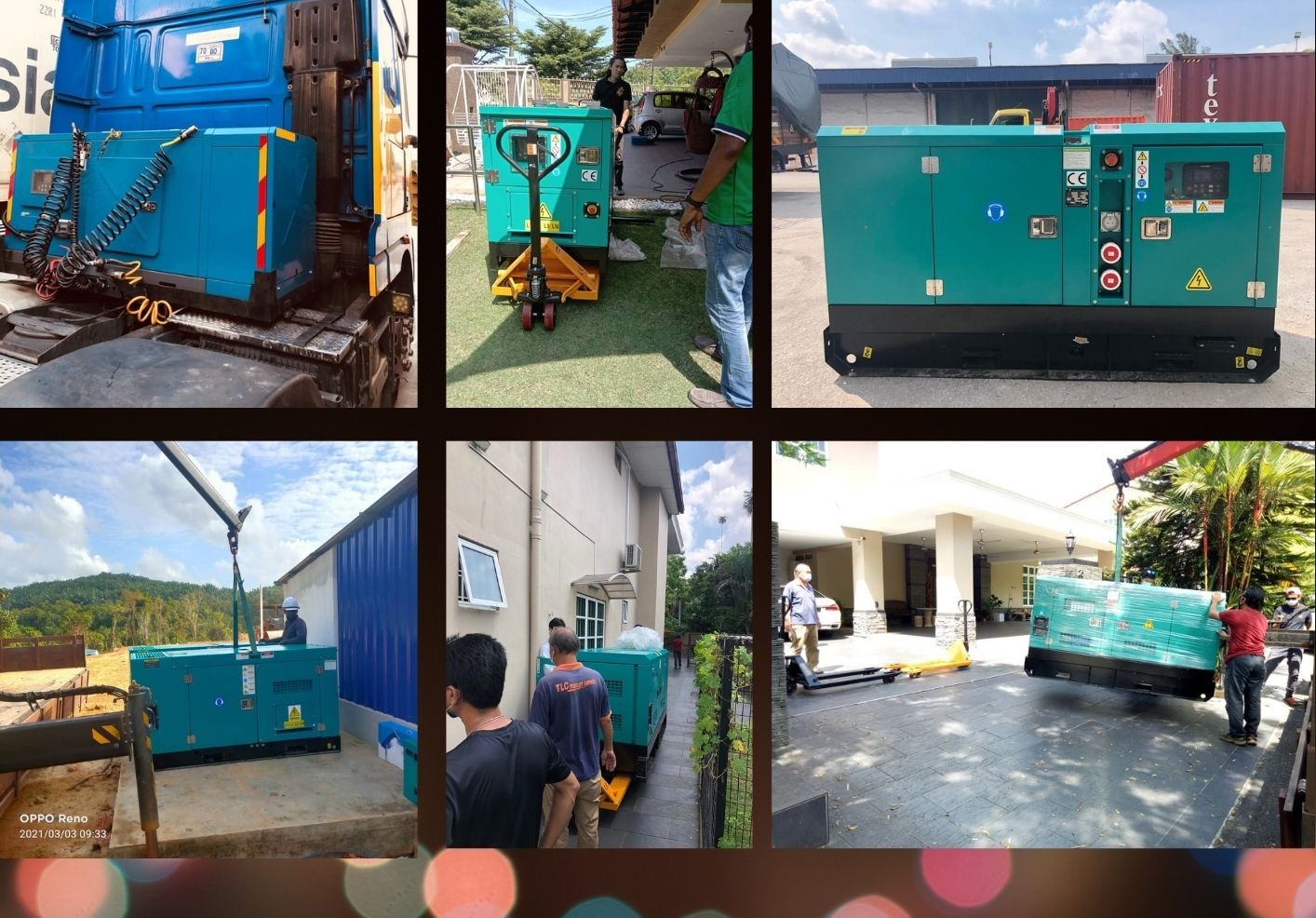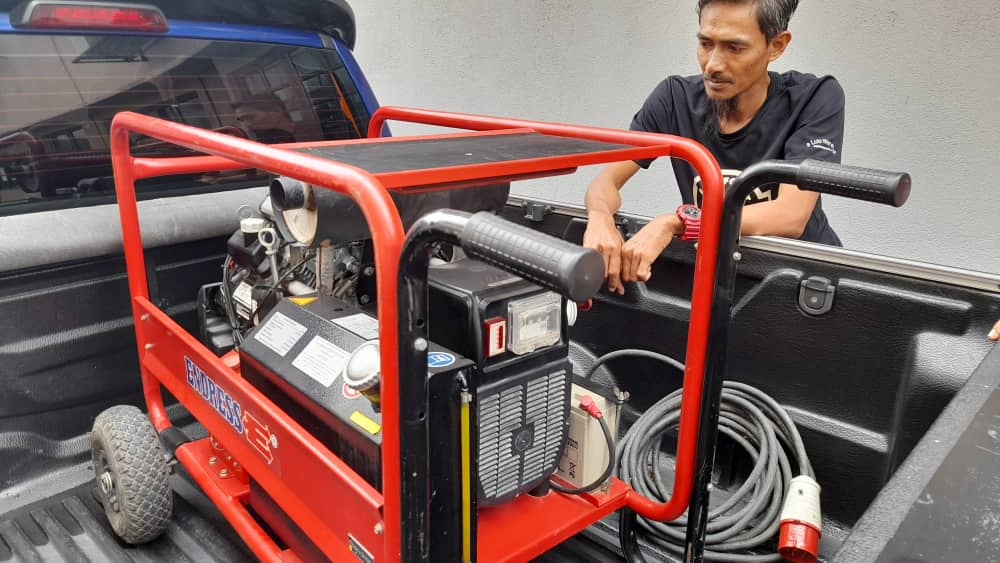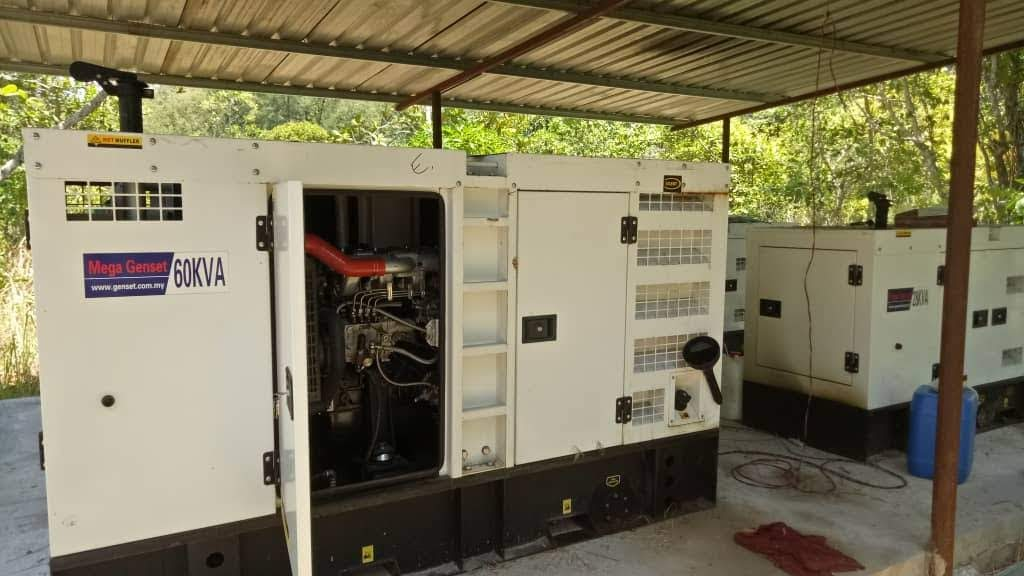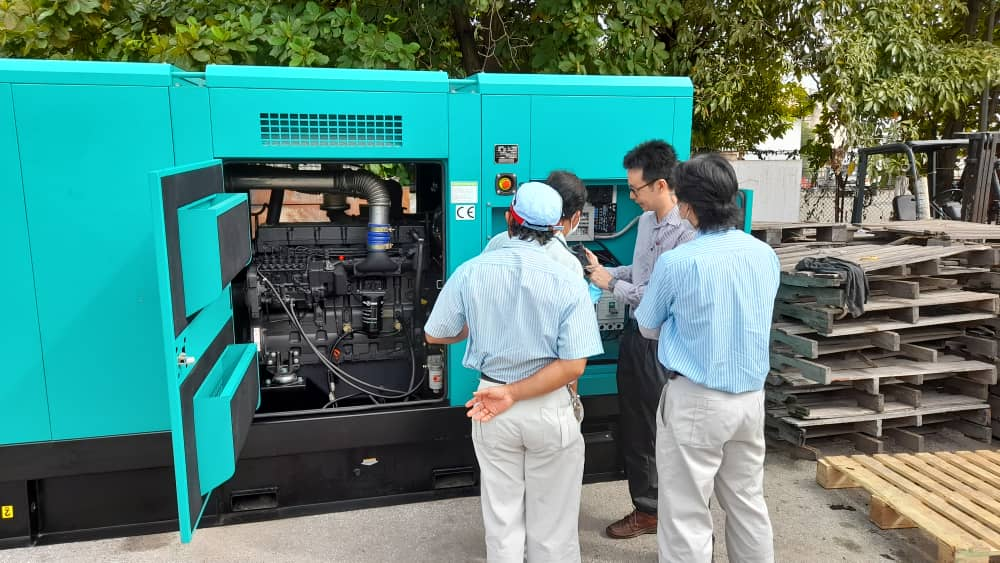### CHOOSING THE RIGHT SIZE GENERATOR

Housing generator provide a backup for your home’s power needs in case of TNB power cut off. The generator can quickly provide the power your home needs in case of an interruption to your regular power grid, whether through automatic generator transfer switches or manual generator transfer switches.### WHAT SIZE GENERATOR DO YOU NEED?

Calculate the electric applicants you want to power. Check the product’s manual to determine how much wattage the device needs to run. Multiply the running wattage of the device by three. Add the running wattage to the starting wattage for the total wattage the device needs to operate.  For example, a small refrigerator uses about 350 watts to run, so it’s starting wattage would be around 1050 watts, or three times its running wattage. Therefore, the total wattage you need to run a small refrigerator is about 1350 watts. In this case, you will need a generator with minimum of 1000 to 2000 watts. Written out formula form will be : (Running wattage x 3) + Starting wattage = Total wattage needed.### HOW TO CALCULATE WATTS TO AMPS

If you’re thinking “what size of a generator do I need?” what you really want to know is how much generator power or total wattage you need. To determine the best generators for home use. Add all your wattage numbers together. This will give you a rough wattage usage. For example, you might need something as small as a 2000-watt inverter generator or a couple of 5000-watt generators.

Converting watts to amps can be done using the power formula, which states that I = P ÷ E, where P is power measured in watts, I is current measured in amps, and E is voltage measured in volts. Therefore the current I in amps is equal to the power P in watts divided by the voltage V in volts.

Amps = Watts ÷ Voltage
1 Amps = 1 kVA